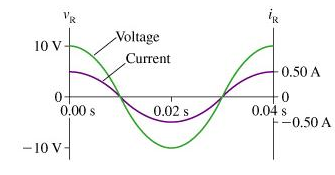# Problem: The figure shows voltage and current graphs for a resistor.Part AWhat is the value of the resistance R? Express your answer using two significant figures.R = _______ΩPart BWhat is the emf frequency f?Express your answer using two significant figures.f = __________Hz

###### FREE Expert Solution

$\overline{){\mathbf{V}}{\mathbf{=}}{\mathbf{IR}}}$

$\overline{){\mathbit{f}}{\mathbf{=}}\frac{\mathbf{1}}{\mathbf{T}}}$

Part A

At t = 0s, V = 10 V and I = 0.5 A.###### Problem DetailsThe figure shows voltage and current graphs for a resistor.

Part A

What is the value of the resistance R?

R = _______Ω

Part B

What is the emf frequency f?